deutsch     english    français     Print

## 2.8 WHILE LOOPS### INTRODUCTION

 You have already gotten to know the command repeat, with which you can repeat a program block several times. However, it is important to know that you can only use repeat this way in TigerJython and not in other Python flavours. On the other hand, you can use the while structure everywhere. The while loop is initiated with the keyword while, followed by a looping condition. The instructions in the loop block are repeated as long as the condition is fulfilled. The program then continues on with the next statement listed after the loop block. PROGRAMMING CONCEPTS: Iteration, while structure, combined conditions, loop termination

### SPIDER WEB

 The turtle should draw a rectangular spiral with the help of a while loop. We will use a variable a, with an initial value 5which is then increased by 2 with each iteration of the loop. As long as the condition a < 200 is true, the statements in the loop block will be executed. To make it a bit more fun, you can switch out the turtle icon for a spider.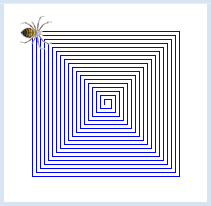```from gturtle import *

makeTurtle("sprites/spider.png")

a = 5
while a < 200:
forward(a)
right(90)
a = a + 2
```
Highlight program code (Ctrl+C copy, Ctrl+V paste)

### MEMO

 A while loop is used to repeat a program block. In order for the program block to be executed, the condition must be true. Because of this, one might also call it a "running condition". If the change in value is missing in the loop block, the running condition always stays true and the program remains endlessly "hanging" in the loop. In our learning environment, you can cancel the hanging program with the stop button or by closing the turtle window. In general, infinite loops without a the option to cancel are dangerous, and in an extreme case you will have to reboot your computer.

### COMBINING CONDITIONS WITH OR

 The turtle should draw the adjacent figure using the while loop. As you can see, it is drawn with alternating red and green triangles. You can use the following trick to change the colors: Test the loop variable to see whether it is 0, 2 or 4 and then choose the pen color red. Using the command fillToPoint(0. 0) you can fill a figure with color while drawing. In this case, it is as though a rubber band was attached to the point (0, 0), the other end of which the turtle drags along. All points the rubber band reaches along the way are colored consecutively.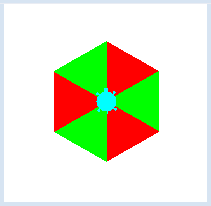```from gturtle import *

def triangle():
repeat 3:
forward(100)
right(120)

makeTurtle()
i = 0
while i < 6:
if i == 0 or i == 2 or i == 4:
setPenColor("red")
else:
setPenColor("green")

fillToPoint(0, 0)
triangle()
right(60)
i = i + 1
```
Highlight program code (Ctrl+C copy, Ctrl+V paste)

### MEMO

 You must pay attention to the correct indentation for each loop block when using several program structures. As you can see, you can combine two or more conditions using or. A condition linked this way is true if either of the conditions are satisfied, and it is also true if both conditions are met. Using the command fillToPoint(x, y) you can fill figures with the pen color while drawing, as opposed to the command fill(), with which you can fill already drawn closed figures.

### COMBINING CONDITIONS WITH AND

 The turtle should draw 10 connected houses using a while loop. The houses are numbered from 1 to 10. The houses with the numbers 4-7 are large, and all of the other houses are small. In the while loop, the house number nr is used to determine the size of the houses. The houses are large if nr is greater than 3 and less than 8. We use the command fillToHorizontal(0) to add color. As a result, the area between the drawn figure and the horizontal line y = 0 is filled consecutively.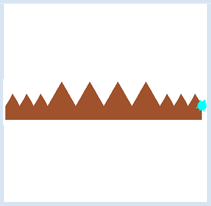```from gturtle import *

makeTurtle()
setPos(-200, 30)
right(30)
fillToHorizontal(0)
setPenColor("sienna")

nr = 1
while nr <= 10:
if nr > 3 and nr < 8:
forward(60)
right(120)
forward(60)
left(120)
else:
forward(30)
right(120)
forward(30)
left(120)

nr += 1
```
Highlight program code (Ctrl+C copy, Ctrl+V paste)

### MEMO

 You can link two conditions with and. Such a linked statement is only true if both conditions are met. Using the command fillToHorizontal(y) you can fill figures with the pen color while drawing. This way, the area between the drawn figure and the horizontal line at y  is filled. nr += 1 means that nr is increased by 1. It is just an abbreviation for the assignment nr = nr + 1.

### EXITING LOOPS WITH BREAK

 A loop whose condition is always true will loop forever. However, you can force a loop to exit at any time using the keyword break. Your program will draw rotated squares with increasing side lengths until the side length is 120.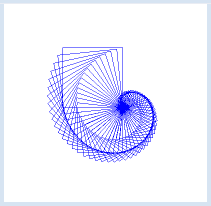```from gturtle import *

def square(sidelength):
repeat 4:
forward(sidelength)
left(90)

makeTurtle()
hideTurtle()

i = 0
while True:
if i > 120:
break
square(i)
right(6)
i += 2
print("i =", i)```
Highlight program code (Ctrl+C copy, Ctrl+V paste)

### MEMO

 With a while True loop, a program block can be repeated, until the program is aborted by closing the turtle window. The loop is run in increments of two. Instead of using i = i + 2 you should use the abbreviated notion i += 2 (i is incremented by 2). With the command print-you can write something into the TigerJython console at the bottom of the editor. With text you should use quotation marks and numbers should be separated by a comma. A space will automatically be inserted between the text and the number. Do you understand why the output is i = 122? The keyword continue is rarely used. It skips the remaining part of the body of the loop.

### INPUT VALIDATION

 If you ask the user to enter a number restricted to a certain range, you cannot trust him that he adheres to your restriction. A "robust" program checks the input and intercepts an incorrect entry with a feedback. This input validation is most easily performed in a while loop which is repeated until the input value is accepted. In your program the user enters the number 1, 2, or 3 to select one the  colors red, green, or yellow of the filled circle. ```from gturtle import * makeTurtle() n = 0 while n < 1 or n > 3: n = inputInt("Enter 1, 2 or 3") if n == 1: setPenColor("red") elif n == 2: setPenColor("green") else: setPenColor("yellow") dot(200) ``` Highlight program code (Ctrl+C copy, Ctrl+V paste)

### EXERCISES

1.

 The turtle moves forward on a line with length 5 and turns 70° to the right. Then it increases the line length by 0.5 and repeats these steps as long as the line length is smaller than 150. Try it also with the rotation angle of 89 °!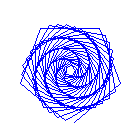2.

 As you probably noticed, the rotation angle at a tip of the 5 edged star is 144°. Change this rotation angle by just a little bit, for example to 143°, and increase the number of repetitions. You will then get a new figure.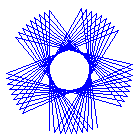3.

 The turtle draws a diagonal pattern with filled red circles. All circles are located in the Turtle window, which means that the distance from the center is less than 400. Use the command dot(25) for creating the circles.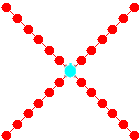4.

 The turtle is located at the position (250, 200). With steps of length 10, the turtle moves on a straight line to the home position until the distance is less than 1. Use the commands towards() and heading(degrees) (see documentation).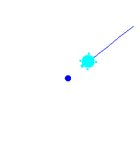5*.

 In order to stop the turtle more precisely at home, you decrease the distance by a factor 10 to 100. Unfortunately it may happen that the turtle does not stop anymore. Can you explain this behavior?